# Proof assistant

For a mathematical theory, correctness means formalizability. "In practice, the mathematician ... is content to bring the exposition to a point where his experience and mathematical flair tell him that translation into formal language would be no more than an exercise of patience (though doubtless a very tedious one)." Reliability of these experience and flair appears to be high but not perfect. Formalization is especially desirable in complicated cases, but feasible only in very simple cases, unless computers help. (Similarly, without computers a programmer is able to debug only very simple programs.)

A proof assistant is a computer program used interactively for developing human-readable reliable mathematical documents in a formal language. (It is not the same as a non-interactive, fully automated theorem prover.)

## Isabelle/Isar

• Isabelle, a generic system for implementing logical formalisms;
• Isar (Intelligible SemiAutomated Reasoning), a versatile language environment for structured formal proofs;
• Proof General, a configurable user interface (front-end) for proof assistants;
• HOL (Higher-Order Logic).

### Example session

#### First impressions

An existing file, verified before, is used as the source text in this example. Thus, the session is not really interactive. However, this fact is not known to Isabelle. The proof assistant treats the session as interactive, and the text as new.

We start Proof General (Fig. 1) and enter the source text (Fig. 2).

On this stage Proof General does not send the text to Isabelle; we still can edit the text. Proof General helps us by automatic colorization according to the syntax of the Isar language.

We start a new theory "CauchysMeanTheorem". (The well-know Cauchy's Mean Theorem says that the geometric mean is less than, or equal to, the arithmetic mean, for every finite collection of positive numbers. However, this theorem will appear much later, near the end of the source text.) The existing theory "Complex_Main" is a prerequisite. (It contains main facts about real and complex numbers; only real numbers are relevant, but some useful formulas about (a + b)2 appear in "Complex_Main".)

Clicking the red button "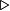$\triangleright$" we send the first portion of the text to Isabelle. After two more such clicks Isabelle reads the line "imports Complex_Main" and finds in the library the "Complex_Main" theory (since it is a prerequisite to the new "CauchysMeanTheorem"), but also "Real", since it appears to be a prerequisite to "Complex_Main", and so on, recursively, until all prerequisites are found and processed (in a logical order). Then, after 9 more clicks we come to the screen shown on Fig. 3. The definitions (and everything before them) are already processed by Isabelle; accordingly, they turn into blue, and become read-only. The formulation of the first lemma is being processed by Isabelle; accordingly, it turns into orange. A fraction of a second later we get Fig. 4. The formulation of the lemma is read by Isabelle; a dialog about its proof starts on the bottom window.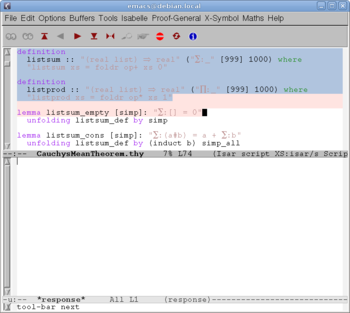Fig. 3: Definitions are done; now reading the lemma.

#### A robot as a student

The first lemma "listsum_empty" (of the new "CauchysMeanTheorem" theory) is ridiculously trivial; it claims that the sum of the empty collection of numbers is equal to zero! Here is an explanation of this disturbing situation.

A human student would not start to learn Cauchy's mean theorem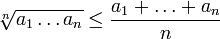$\sqrt[n]{a_1\dots a_n} \le \frac{a_1+\dots+a_n}n$

being unfamiliar with such notions as the sum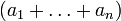$(a_1+\dots+a_n)$ and the product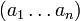$(a_1\dots a_n)$ of n numbers. However, a robot (like Isabelle) is not a human. A robot never was in a kindergarten or elementary school. We cannot say to Isabelle: "Look, here is a sum of three numbers: 15+5+10=30; note that also 5+10+15=30 etc. Likewise, any finite collection of numbers has its sum." Children proceed from the particular to the general, but proof assistants proceed from the general to the particular.

For now, Isabelle is not well-educated. Its mathematical knowledge is rather fragmentary. Some volunteers contribute some theories to the library of Isabelle; this is not a well-organized process rich of resources. Each contributor has to find out, which of the relevant facts are already known to Isabelle and which are not.

Definitions of the sum and the product of a list of numbers are given to Isabelle just before the first lemma (see "listsum" and "listprod" on Fig. 3 or 4) in terms of

• "foldr", the right fold operation defined in the "List" theory (one of the prerequisites).

Informally, the right fold applies to a binary operation (say, "+"), a list (say, "[x,y,z]") and an initial value (say, "a"), giving "x+(y+(z+a))"; more formally,

foldr op+ [x,y,z] a  =  x+(y+(z+a)).


(For the summation case one may write just "x+y+z+a", but in general an operation need not be associative.) The "listsum" definition stipulates the addition operation and the initial value 0; the "listprod" definition stipulates the multiplication operation and the initial value 1. The sum of a list is denoted by Isabelle as follows:$\Sigma: [x,y,z] = x+(y+(z+0)) \, .$

A human student, given by such a formal definition of sum (only sketched here) would not be too astonished by the trivial exercise "prove that the sum of the empty list is equal to 0"; this is our "listsum_empty" lemma. After the formulation, the source text contains a hint to the proof,

unfolding listsum_def by simp


which means informally: "Isabelle, use the definition of the sum of a list, do evident simplifications, and hopefully you'll find a proof of the lemma." And indeed, Isabelle is cute enough in order take the hint. After a fraction of a second it reports that the lemma is successfully proved.

Now we continue the example session.

#### Hard work

After more than 30 rather boring, mostly trivial lemmas (in a course for human students they probably would be called exercises), whose proofs vary in length from one line to about 50 lines, we arrive to a more interesting lemma (Fig. 5) and start proving it (Fig. 6).

We make a claim of the form "from A have B..."; Isabelle responds by "using this: A, goal: B" (Fig. 7) and waits. We add a hint "...by C"; Isabelle takes the hint, finds a proof and responds by "have B" (Fig. 8).

We continue; the work becomes hard (Fig. 9). After a lot of effort we get close to the happy end (Fig. 10), and finally the theorem is proved (Fig. 11).

#### Options

Many options are available for Proof General (Fig. 12) and Isabelle. In particular, many kinds of additional information may be requested from Isabelle at any moment of the session (Fig. 13).

### Higher-order logic versus theory of sets

The logical structure of a mathematical text is "the choreography of$\forall, \exists, \wedge, \vee$ and$\Longrightarrow$" ; these are symbols for quantifiers ("for all", "exists") and connectives ("and", "or", "implies"). Quantifiers bind variables over needed ranges.

Example 1. Every real number has its qubic root. More formally, for every real number x there exists a real number y such that y3 = x. Symbolically,$\forall x \in \mathbb{R} \; \exists y \in \mathbb{R} \; (y^3=x).$ The range of the quantification is the real line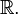$\mathbb{R}.$

Quantifiers with a variable and range, like$\forall x \in \mathbb{R},$ are inconvenient for formal deduction. Usually the range is avoided in one of two ways. One way ("typed variables"): the variables x, y are reserved for real numbers, and the formula becomes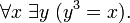$\forall x \; \exists y \; (y^3=x).$ The other way ("guarded quantifiers"): the variables are not reserved, and the formula becomes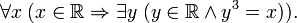$\forall x \; ( x \in \mathbb{R} \Rightarrow \exists y \; ( y \in \mathbb{R} \wedge y^3=x) ).$

Example 2. Every bounded set of real numbers has its least upper bound. More formally: for every subset A of the real line, if there exists a real x greater than, or equal to, all elements of A, then there exists the least such x. The variable A runs over the set of all subsets of the real line.

Example 3. Every continuous map (that is, function) from a circle to a sphere can be extended to a continuous map from the disk (bounded by the circle) to the sphere. Here, variables run over maps.

We observe that the range of quantification is sometimes a given set X, sometimes the set YX of all maps from one given set X to another given set Y, and in general a set that results from several given sets by a finite number of such operations. Using typed variables we get accordingly first-order logic, second-order logic, and higher-order logic. For instance, third-order logic appears when dealing with all topologies on X. Subsets of X may be treated as maps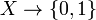$X \to \{0,1\}$; sequences of points of X may be treated as maps$\{1,2,\dots\} \to X.$

Using Isabelle/HOL syntax, Example 1 may be written as "$\forall$x::real.$\exists$y::real. y^3=x", which is of first order. Second-order examples: "for every subset A of the real line" may be written as "$\forall$A::real set", while "for every function f from the real line to itself" may be written as "$\forall$f::real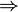$\Rightarrow$real". Crucial notions "map" and "set" are ingrained in HOL, which simplifies formulations and proofs, thus making the proof assistant more efficient.

Alternatively, one may use untyped variables and express everything in the framework of set theory by means of guarded quantification. In this case maps and sets are reduced to the binary relation "$\in$" (membership) external to the logic. The proof assistant cannot provide any special service for them.

Example 4. Every linear space has a basis. Here, one variable runs over the class of all linear spaces, and another variable - over the set of all subsets of the linear space.

The class of all linear spaces cannot be treated as a (given) set. It may seem that we must leave the higher-order logic for the theory of sets. However, we may redesign the theory of linear spaces as a theory with the axiom "L is a linear space", and the theorem as "L has a basis". Now only one variable is bound by a quantifier, and it runs over L. The quantifier "for every linear space L" becomes implicit; the theory holds for all linear spaces.

During the 20 century the theory of sets was used as a universal infrastructure behind various specialized mathematical theories. The higher-order logic is weaker than the theory of sets, but still strong enough in order to accommodate the specialized theories. For those preferring the habitual set-theoretic framework, Isabelle (together with Isar and Proof General) provides the option to use the logic ZF (Zermelo-Frenkel theory of sets) instead of HOL (higher-order logic). This option is used in IsarMathLib, a stand-alone library of formalized mathematics for Isabelle/Isar/ZF. Here is a quotation from "Questions and Answers" of IsarMathLib (see the external links page):

Q3: Why ZF logic?
A: Almost all mathematics I know about is based on the first order logic and Zermelo-Fraenkel set theory. This is what I was taught in high school and feels natural to me. I am sure other logical frameworks, like HOL, are interesting and useful, but someone would have to pay me money to make me twist my brain around HOL and its typed set theory.

Comparing IsarMathLib with the HOL library it is easy to note that HOL makes the formal text shorter, simpler, and more elegant than ZF. And no wonder: guarded quantification evidently is more cumbersome than typed variables. Isabelle/ZF leaves idle not only the helpful mechanism of types but also some advanced tools of Isabelle/HOL (arithmetic decision procedures, records, locales etc). In the long run this loss should outweigh the burden of initial learning again.

Specialized mathematical theories are usually formulated in terms of mathematical structures, — spaces of various kind (linear, topological etc) and algebraic structures (groups, rings, fields etc). A unified theory of structures introduces a scale of sets. The first level is occupied by a base set, or several base sets. Such sets as 2X (the set of all subsets of a base set X) occupy the second level. And so on.

The evident similarity between these scales of sets and the higher-order logic opens the door to a natural treatment of mathematical structures in HOL. To this end Isabelle/Isar/HOL stipulates so-called locales and type classes.

Here is an example borrowed from the "RealVector" theory of the Isabelle/HOL library. The mathematical definition of a vector space over a field is formalized as a locale:

locale vector space =
fixes scale :: " 'a::field => 'b::ab_group_add => 'b"
assumes
"scale a (x+y) = scale a x + scale a y"
and
"scale (a+b) x = scale a x + scale b x"
and  "scale a (scale b x) = scale (a$\ast$b) x"
and  "scale 1 x = x"

Some comments (for those acquainted with vector space). The scalar multiplication is denoted by scale; thus, "scale a x" is what mathematicians write just "ax". The first argument belongs to a field, the second to an abelian group ("ab_group_add") written additively (thus, "x+y" is the sum of x and y), and the result belongs to the same group. It is assumed that vector addition is distributive over scalar multiplication: a (x+y) = a x + a y; three more assumptions follow.

Such a close contact between mathematical content and proof assistant, provided by Isabelle/HOL, cannot be provided by Isabelle/ZF.

### Reliability

Formal languages inevitably are less human readable than natural languages. However, some formal languages are less human readable than other formal languages. These are low-level languages. Being simple they are convenient for theoretical analysis and automatic processing. Being poor they are inconvenient for expressing human thoughts. To this end one needs high level languages, rich of means of expression. (This situation is observed in logic and programming as well.)

The high-level Isabelle/Isar/HOL formal language is supported by a complicated software. Such a software usually contains some bugs. However it does not mean that the proof assistant sometimes certifies wrong theorems, as explained below.

Isabelle contains a relatively small, simple, trustworthy component, the kernel. Only the kernel can certify a theorem. The kernel does not understand the high-level language, but only a low-level ("primitive") language. Other components of Isabelle translate proofs from the high-level language into the low-level language and sumbit them to the kernel. An error outside the kernel cannot force the kernel to accept a wrong proof.

Still, some doubts remain. Execution of the kernel involves a hardware, an operating system, and an implementation of a programming language. These can be buggy,corrupted by a hacker, etc.; but such scenarios are rather exotic.

A more realistic scenario is an incorrect translation into the primitive language. It may happen that a (right) theorem certified by the kernel is not equivalent to a (wrong) "theorem" written and read by humans. It is also noted that the kernel is not so much small and simple as to be very trustworthy.

A higher reliability can be achieved by an independent proof checker, a very small and simple program, possibly executed on a different computer, intended solely for verification of proofs translated by a proof assistant into a low-level language. But still, this translation process escapes verification.

A human author, interacting with the proof assistant, creates a new theory on top of old theories, typically created by others. In contrast to the proof assistant, the author usually does not investigate scrupulously all the definitions in the old theories. Rather, the new author assumes that the predecessors did not deviate inadvertently from the mathematical traditions. This is another potential source of errors: the theorem meant by the author can differ from the theorem certified by Isabelle.

### Availability

Isabelle is available to download (see the external links page), free of charge or registration. For users of Linux or MacOSX the installation is quite easy; users of Windows need Cygwin, a Unix/Linux-like environment for Windows. Everything is included in the bundle: Isabelle, Isar, Proof General, HOL and more, as well as documentation and libraries of theory files.

For especially interested, the source code is also available; it contains mostly programs in the functional programming language ML.

Isabelle for Linux, version 2009-2, is distributed as a file "Isabelle2009-2_bundle_x86-linux.tar.gz", 247 MB long. Unpacking it one gets several folders (bin, contrib, doc, etc, heaps, lib, src), 724 MB in the total. The file "bin/isabelle" is a bash script to be called by the command "isabelle emacs" in order to start Proof General. (No compilation or installation needed.)

The "src/HOL" folder contains 90 theory files (such as "Complex_Main", "Deriv" and "Complete_Lattice") and 48 folders (such as "Algebra", "Hahn_Banach", "Matrix" and "Probability"), about 900 theory files in the total.

The "doc" folder contains documentation:

• Learning and using Isabelle
• "tutorial": Tutorial on Isabelle/HOL (Fig. 14, x+213 pp.)
• "main": What's in Main
• "isar-overview": Tutorial on Isar
• "locales": Tutorial on Locales
• "classes": Tutorial on Type Classes
• "functions": Tutorial on Function Definitions
• "codegen": Tutorial on Code Generation
• "nitpick": User's Guide to Nitpick
• "sledgehammer": User's Guide to Sledgehammer
• "sugar": LaTeX Sugar for Isabelle documents
• Reference Manuals
• "isar-ref": The Isabelle/Isar Reference Manual (Fig. 15, vi+207 pp.)
• "implementation":The Isabelle/Isar Implementation Manual
• "system": The Isabelle System Manual

## Other systems

• Mizar
• Hol88, Hol98, Hol4, Hol Light
• Nuprl
• IMPS
• Lego, Coq
• Lambda-CLAM
• Matita
• Prover9
• Mace2
• Jape, Ssreflect, PhoX, PVS, Alfa, Theorema
• ACL2, Twelf, Minlog, Omega